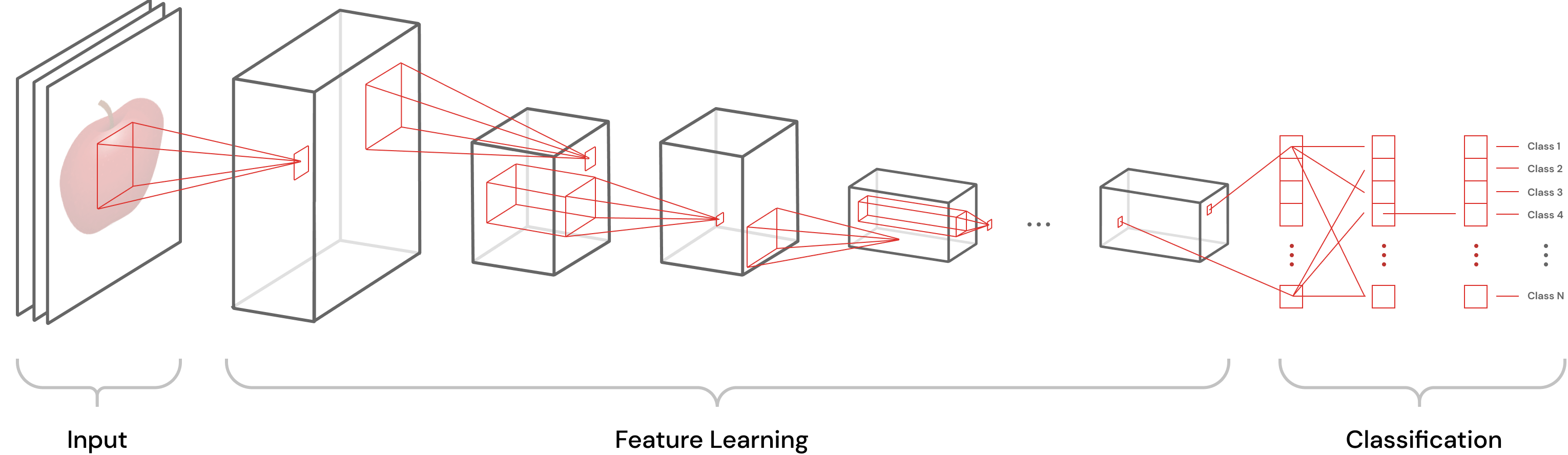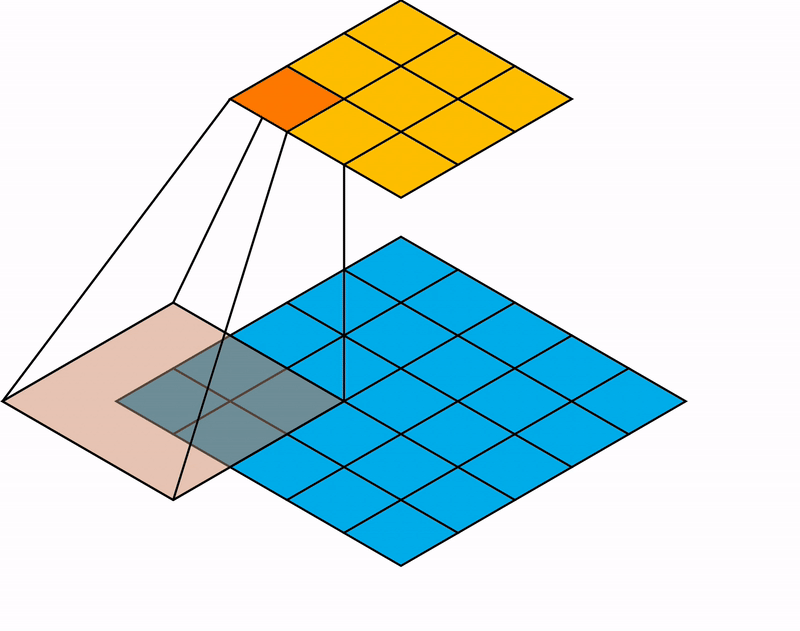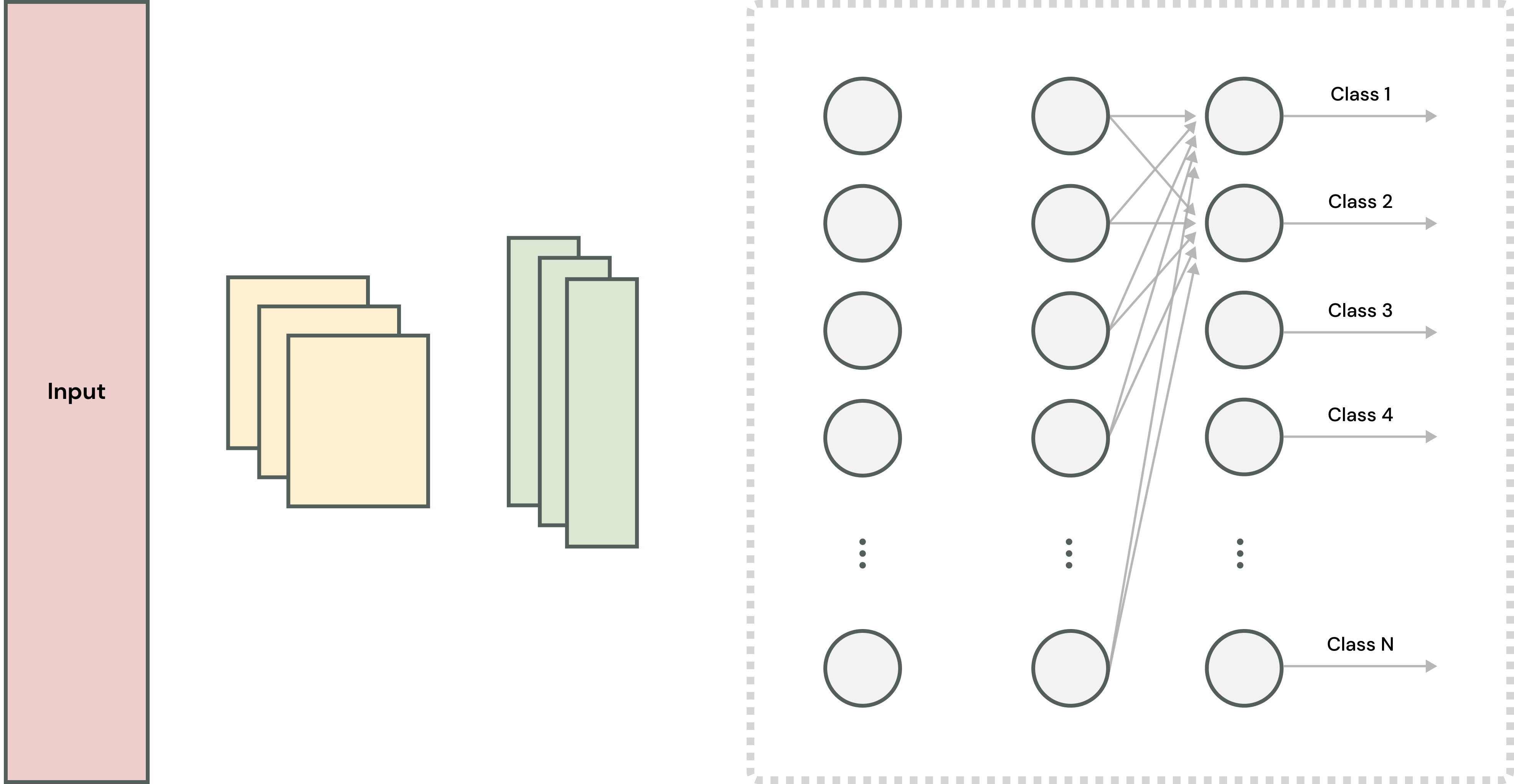# Convolutional Neural Networks: Machine Learning's Eye

##### Technical Consultant

Let’s take a step back and examine a machine learning architecture that has become the go-to for image recognition and computer vision.

Convolutional neural networks (CNN) are the go-to machine learning architectures for image recognition and computer vision. Let’s take some time to understand CNNs. Let’s look at their origins, their architecture, and their workings.

### A Biologically-Inspired Origin

CNNs are a class of deep neural networks that have become synonymous with image recognition and computer vision. They are trainable, multistage machine learning models. They originated from the neocognitron. In 1980, Kunihiko Fukushima built the neocognitron by extending some of his previous work (link). He drew inspiration from the human visual nervous system. The neocognitron is a multilayered neural network capable of unsupervised learning. It learns to recognize stimulus patterns based on the geometrical similarity of their shapes. It is not affected by changes to the position or structure of the stimuli.

Yann Lecunn et al. built upon the basic idea of the neocognitron and, in 1998, proposed the LeNet neural network for optical character recognition of single spectral-channel (i.e., grayscale) images (link). LeNet is a simple, low memory consuming model that is often considered the first true CNN.

### A Breakdown

As stated above, CNNs are multistage, multilayer models that have multiple layers of artificial neurons. Each layer of the CNN has its role. The layers build upon each other to segment, discriminate, and classify the inputs. The CNN achieves a degree of shift and distortion invariance by combining three architectural ideas: local receptive fields, shared weights, and spatial subsampling. Each stage of the CNN takes in and outputs feature maps (i.e., sets of arrays). So, multiple 2D arrays represent a color image. Each array represents the image in one color channel. Each unit of a layer (i.e., neuron) receives input from a small neighborhood of neurons of the previous layer. The neuron in the current layer then extracts features (e.g., edges, corners, heads) from the input feature maps depending on its type. This approach is called local receptive fields. If we imagine a single neuron carrying out this operation, then that neuron would scan each feature map, one at a time. Then, given its local receptive field, the neuron would produce outputs as it scans. It stores those outputs at corresponding locations on the output feature maps. So, the operation is the equivalent of convolving the input image with a smaller-sized kernel and then squashing it.A CNN is a collection of stages that extract and learn features followed by a classification module.

Of course, doing this sequentially for all the pixels of all the color channels of an image would be horrendously time consuming.  So we parallelize this operation by using multiple neurons that share the same weights. In other words, the neurons extract the same features. Collectively, these neurons form a filter. CNNs use many such filters. So, CNNs can successfully capture spatial dependencies.

### Digging a Little Deeper

So, a CNN is, basically, a collection of stages that extract and learn features followed by a classification module. The feature extraction stages are typically composed of a convolution layer, a non-linearity layer, and a pooling layer. The early stages extract low-level features (e.g., edges). Successive stages build upon earlier stages to extract evermore complicated features (e.g., corners).

#### Convolution Layer

Let’s examine the convolution layer. The convolution layer is, basically, a filter bank. It holds various trainable filters that extract different features. The filters are optimized to pull out specific features via gradient descent and backpropagation. The overall CNN also uses gradient descent and backpropagation to train itself. Let’s take a look at an example of how a single filter processes data to understand what is going on.

Let’s assume that we have a typical CNN that processes 2D color images. Also, suppose that the input image has a resolution of 5 ˣ 5 pixels. So, instead of seeing a color image as we do, computers see the image as three 5 ˣ 5 matrices (one matrix for each color of RGB). The value of each cell in the matrices is a number that corresponds to a pixel’s value in a given color band. Upon ingesting the input, the CNN breaks the input matrices into smaller matrices offset by a stride value. For our example CNN, the input matrices are broken up into 3 ˣ 3 matrices offset by a stride value of 2. Then, we convolve the smaller matrices with the filters. So, the filters effectively scan the inputs.The input image is convolved with the filter one block at a time.

The filters are just small matrices that, before training, contain random values. For example, we have a single 3 ˣ 3 filter. When we convolve the filter and the input image, we take each sub-matrix and dot product it with the filter. We place the result of those dot products, numbers, into output matrices at appropriate locations. Given our example CNN, we take the first 3 ˣ 3 block (i.e., sub-matrix) of the input image in a given color band and dot product it with our filter. We place the result of that dot product in the first cell of our output matrix In other words, if O is our output matrix, then we place the number in cell O₁₁. We repeat this process for all 3 ˣ 3 blocks until all blocks are exhausted. So the convolved second block goes into O₁₂, the third goes into O₁₃, and so on. Once complete, O is the convolved input matrix in a color band.

#### Non-Linearity Layer

The non-linearity layer comes after the convolution layer. The non-linearity layer increases the non-linearity of the outputs of the convolution layer. It takes the convolved input matrices, weights them, and runs them through non-linear activation functions to create activation/feature maps. This layer is needed because, up to this point, the CNN has been performing linear computations. However, relationships in our world are typically not linear. So we need to add non-linearity into the system to allow the system to learn non-linear relationships. For example, an edge in a grayscale image may not go from true black (the presence of an edge) to true white (the lack of an edge) immediately. Instead, there may be many shades of gray as black transitions to white. Nevertheless, the system should still recognize the edge as an edge.

While many non-linear functions can be used, the rectified linear unit (i.e., ReLu) is the go-to non-linear activation function nowadays. The advantage of the ReLu, relative to other popular non-linear functions like hyperbolic tangent and sigmoid, is that it does not saturate. In other words, values exceeding the boundary values do not get set to the boundary values (e.g., large numbers snap to 1 and small numbers snap to -1). Because of saturation, the hyperbolic tangent and sigmoid functions are only sensitive in their middle ranges. Additionally, error gradients decrease significantly every time they get propagated. So, error gradients going to layers deep in the network are unuseful (i.e., vanishing gradient). Vanishing gradients are something deep networks need to overcome.

The pooling layer downsamples and summarizes the feature maps from the non-linear layer.

ReLu is a piecewise linear function. For non-negative input values (i.e., x ≥ 0), ReLu just outputs the input value (i.e., y = x). For negative values (i.e., x < 0), ReLu outputs zero (i.e., y = 0). So ReLu looks and acts like a linear function (e.g., it doesn’t saturate), but can still learn complex relationships since it is a non-linear function. The equation for ReLu is simple enough:

f(x) = max(0, x)

Unfortunately, ReLu is non-differentiable at the origin (i.e., 0). So we instead use a smoothed version of it called the Softplus:

f(x) = ln(1 + eˣ)

#### Pooling Layer

Following the non-linear layer is the pooling layer. The pooling layer downsamples and summarizes the feature maps from the non-linear layer. All subsequent processing uses these summaries. So, the pooling layer decreases the number of parameters that have to be learned by the CNN. Consequently, the computational overhead decreases.

The user selects the pooling window size and a pooling methodology to set up the pooling layer. For our example CNN, we will use a 3 ˣ 3 pooling window and the maximum pooling methodology. So, for each feature map from the non-linearity layer, we will apply the selected pooling methodology to it, corresponding to our pooling window size. Since our pooling window is the same size as the feature map from the non-linearity layer, the process is easy enough. We consider all the values in the feature map when applying our pooling method. In other words, we choose the maximum value in the feature map.

#### Classification Module

The last CNN component is the classification module or the fully-connected layer. The module learns to classify the inputs. For example, if a CNN is optimized to recognize cats, the layers preceding the classification module extract relevant features. The layers build on top of preceding layers and pull out increasingly complex image features. Simply put, the CNN may first extract edges, then ears, then a head. The classification module is where the CNN learns to differentiate a cat from another animal, like a dog.The fully-connected layer takes the downsampled feature maps and uses them to classify the input.

Structurally, this module is similar to a typical feedforward network. It takes in the summarized feature maps from the pooling layer and runs them through a fully-connected neural network. After the data has passed through all the hidden layers, the module runs the product of its output layer through a softmax, getting the input's category.

Banner image credit to
rangizzz
Neural Networks